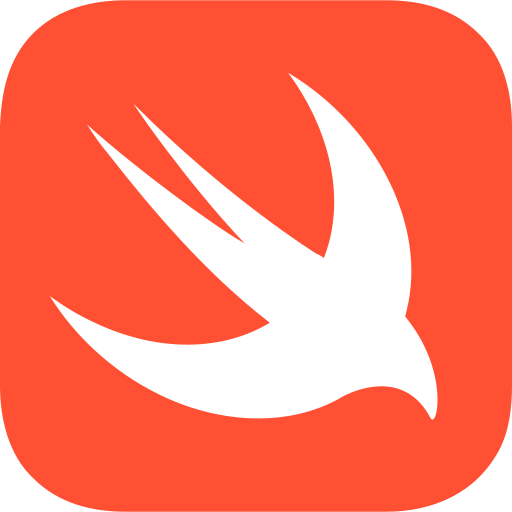# AI coding assistant for busy programmers

## Determine the factors of a number. in Swift## 🤖 Code Explanation

func isPrime(_ number: Int) -> Bool {
// A prime number is a number that is greater than 1 and
// has no positive divisors other than 1 and itself.

// Check if the number is greater than 1
guard number > 1 else {
// If the number is less than or equal to 1, it is not prime
return false
}

// Check if the number is evenly divisible by any number between 2 and itself
for i in 2.. if number % i == 0 {
// If the number is evenly divisible by any number between 2 and itself, it is not prime
return false
}
}

// If the number is not evenly divisible by any number between 2 and itself, it is prime
return true
}